International
Tables for
Crystallography
Volume D
Physical properties of crystals
Edited by A. Authier

International Tables for Crystallography (2006). Vol. D, ch. 1.1, pp. 3-4

## Section 1.1.1.2. Notion of tensor in physics

A. Authiera*

aInstitut de Minéralogie et de la Physique des Milieux Condensés, Bâtiment 7, 140 rue de Lourmel, 75015 Paris, France
Correspondence e-mail: aauthier@wanadoo.fr

#### 1.1.1.2. Notion of tensor in physics

| top | pdf |

Each of the quantities mentioned in the preceding sectionis represented by a mathematical expression. Some are direction independent and are represented by scalars: specific mass, specific heat, volume, pressure, entropy, temperature, quantity of electricity, electric potential. Others are direction dependent and are represented by vectors: force, electric field, electric displacement, the gradient of a scalar quantity. Still others cannot be represented by scalars or vectors and are represented by more complicated mathematical expressions. Magnetic quantities are represented by axial vectors (or pseudovectors), which are a particular kind of tensor (see Section 1.1.4.5.3). A few examples will show the necessity of using tensors in physics and Section 1.1.3will present elementary mathematical properties of tensors.

 (i) Thermal expansion. In an isotropic medium, thermal expansion is represented by a single number, a scalar, but this is not the case in an anisotropic medium: a sphere cut in an anisotropic medium becomes an ellipsoid when the temperature is varied and thermal expansion can no longer be represented by a single number. It is actually represented by a tensor of rank 2. (ii) Dielectric constant. In an isotropic medium of a perfect dielectric we can write, in SI units,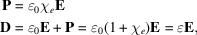where P is the electric polarization (= dipole moment per unit volume),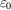the permittivity of vacuum,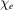the dielectric susceptibility, D the electric displacement andthe dielectric constant, also called dielectric permittivity. These expressions indicate that the electric field, on the one hand, and polarization and displacement, on the other hand, are linearly related. In the general case of an anisotropic medium, this is no longer true and one must write expressions indicating that the components of the displacement are linearly related to the components of the field: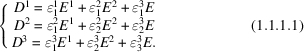The dielectric constant is now characterized by a set of nine components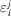; they are the components of a tensor of rank 2. It will be seen in Section 1.1.4.5.2.1that this tensor is symmetric (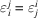) and that the number of independent components is equal to six. (iii) Stressed rod (Hooke's law). If one pulls a rod of length ℓ and cross sectionwith a force F, its length is increased by a quantity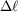given by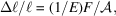where E is Young's modulus, or elastic stiffness (see Section 1.3.3.1). But, at the same time, the radius, r, decreases by Δr given by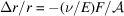, where ν is Poisson's ratio (Section 1.3.3.4.3). It can be seen that a scalar is not sufficient to describe the elastic deformation of a material, even if it is isotropic. The number of independent components depends on the symmetry of the medium and it will be seen that they are the components of a tensor of rank 4. It was precisely to describe the properties of elasticity by a mathematical expression that the notion of a tensor was introduced in physics by W. Voigt in the 19th century (Voigt, 1910) and by L. Brillouin in the first half of the 20th century (Brillouin, 1949). (iv) Expansion in Taylor series of a field of vectors. Let us consider a field of vectors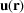where r is a position vector. The Taylor expansion of its components is given by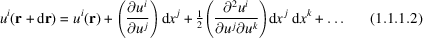using the so-called Einstein convention, which implies that there is automatically a summation each time the same index appears twice, once as a superscript and once as a subscript. This index is called a dummy index. It will be shown in Section 1.1.3.8that the nine partial differentials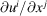and the 27 partial differentials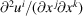are the components of tensors of rank 2 and 3, respectively.

Remark. Of the four examples given above, the first three (thermal expansion, dielectric constant, stressed rod) are related to physical property tensors (also called material tensors), which are characteristic of the medium and whose components have the same value everywhere in the medium if the latter is homogeneous, while the fourth one (expansion in Taylor series of a field of vectors) is related to a field tensor whose components vary at every point of the medium. This is the case, for instance, for the strain and for the stress tensors (see Sections 1.3.1and 1.3.2).

### References

Brillouin, L. (1949). Les tenseurs en mécanique et en élasticité. Paris: Masson & Cie.
Voigt, W. (1910). Lehrbuch der Kristallphysik. Leipzig: Teubner. 2nd ed. (1929); photorep. (1966). New York: Johnson Reprint Corp. and Leipzig: Teubner.